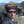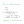0%
0%

# TRCM

Attempt to reinvent the wheel of HDL

Similar projects worth following
219 views
TRCM is new simple, but powerful approach to hardware simulation and synthesis that covers both binary world and ternary world. Started in August 2018 as a library of C++ templates...

Now it's currently developed as simulation of electronics systems with any-bit and any-trit numbers using modern C++ (2011 and above).

Later TRCM also could have:

- converter from Verilog to C++ for faster simulation/verification with C++ "testbench";

- converter from C++ representation to VHDL to synthesize it for your favorite FPGA/CPLD;

- converter from Logisim to C++ for fun etc.

Main idea was to stop using dedicated wires for inputs or outputs in high-level simulation - wires are just wires - each of them could be input or could be output or could be disconnected during simulation. So TRCM (full name is TRCMath C++ library) defines 10 states of the wire to cover all possible scenarios for binary and ternary digital electronics (revised on August 18th 2018):

```// Wire states:
const char TRUE     = 'P'; /* connected to positive voltage */
const char MAYBE    = 'O'; /* connected to intermediate voltage */
const char FALSE    = 'N'; /* connected to the ground */
const char ANYBIT   = 'X'; /* for comparisons only */
const char ANYTRIT  = 'Y'; /* for comparisons only */
const char NC       = 'Z'; /* not connected (high impedance) */
const char PULLUP   = '1'; /* weak pull-up to positive voltage */
const char PULLDOWN = '0'; /* weak pull-down to the ground */
const char PULLMID  = '-'; /* weak pull-middle to intermediate voltage */
const char INVALID  = '?'; /* conflict on the wire (simulation halts) */

// Aliases:
const char HIGHIMP  = NC;
const char POSITIVE = TRUE;
const char NEUTRAL  = MAYBE;
const char NEGATIVE = FALSE;
```

Also TRCM gives user ability to use N-bit or N-trit numbers and perform math with them. Currently available classes:

Signal - the same as Wire<1>;

Wire<N> - N wires;

Uint<N> - N-bit unsigned integer;

Sint<N> - N-bit signed integer;

Tint<N> - N-trit balanced ternary integer (sign is already embedded into this representation naturally).

A few existing TRCM classes support simulation: System, Entity, Connection and RuntimeException.

Source code is available on GitLab under GPL v3: https://gitlab.com/ternary/trcm

And I will post examples of TRCM usage here in this projects as logs...

• ### Simulation of 8-bit increment

This is test3.cpp program:

```#include <TRCMath.hpp>
#undef DEBUG

#include <time.h>
#include <stdio.h>
#include <stdlib.h>

using namespace std;

using namespace TRC;

{
protected:

// indecies:
int iA,iB,iS,iC;

// inputs:
Signal A,B;

// outputs:
Signal S,C;

public:

{

// empty constructor for generic unit

}

void step()
{

if(A==TRUE && B==TRUE)
S = FALSE;
else if(A==FALSE && B==TRUE)
S = TRUE;
else if(A==TRUE && B==FALSE)
S = TRUE;
else // if(A==FALSE && B==FALSE)
S = FALSE;

if(A==TRUE && B==TRUE)
C = TRUE;
else
C = FALSE;

//    cout << name() << ":" << A << B << "->" << C << S << endl;

io(iS) << S;
io(iC) << C;
}
};

class World : public Entity
{

// indecies:
int i_increment,i_input,i_output,i_carry;

public:

// internal counter:
long long counter;

World() : Entity("World")
{
i_increment = at("INC");
i_input     = at("I",8);
i_output    = at("O",8);
i_carry     = at("COUT");

counter = 0;
}

void step() // World works backwards - read outputs and write inputs
{
Wire<9> vec; // temporary vector
#ifdef DEBUG
cout << "Case " << counter << " output=" << vec.binarize() << endl;
#endif

io(i_increment) << TRUE; /* below we change inputs every 16 steps */
io(i_input+0)   << ((counter&(1<<4))?TRUE:FALSE);
io(i_input+1)   << ((counter&(1<<5))?TRUE:FALSE);
io(i_input+2)   << ((counter&(1<<6))?TRUE:FALSE);
io(i_input+3)   << ((counter&(1<<7))?TRUE:FALSE);
io(i_input+4)   << ((counter&(1<<8))?TRUE:FALSE);
io(i_input+5)   << ((counter&(1<<9))?TRUE:FALSE);
io(i_input+6)   << ((counter&(1<<10))?TRUE:FALSE);
io(i_input+7)   << ((counter&(1<<11))?TRUE:FALSE);

counter++;
}

};

unsigned char BYTE = 0;

int main()
{
System *sys = System::getInstance();

World world;

iA = at("INC");
iB = at("I");
iS = at("O");
iC = at("C0");
NAMED(ha0);

iA = at("C0");
iB = at("I");
iS = at("O");
iC = at("C1");
NAMED(ha1);

iA = at("C1");
iB = at("I");
iS = at("O");
iC = at("C2");
NAMED(ha2);

iA = at("C2");
iB = at("I");
iS = at("O");
iC = at("C3");
NAMED(ha3);

iA = at("C3");
iB = at("I");
iS = at("O");
iC = at("C4");
NAMED(ha4);

iA = at("C4");
iB = at("I");
iS = at("O");
iC = at("C5");
NAMED(ha5);

iA = at("C5");
iB = at("I");
iS = at("O");
iC = at("C6");
NAMED(ha6);

iA = at("C6");
iB = at("I");
iS = at("O");
iC = at("COUT");
NAMED(ha7);

unsigned long t1,t2;
int i,n = 100000000;
t1 = clock();
for(i=0;i<n;i++)
{
BYTE++;
BYTE++;
BYTE++;
BYTE++;
BYTE++;
BYTE++;
BYTE++;
BYTE++;
BYTE++;
BYTE++;
}
t2 = clock();
printf("BYTE=0x%2.2X (%6.6fs or %2.2fns per increment)\n",BYTE,
(double)(t2-t1)/CLOCKS_PER_SEC,
(double)(t2-t1)/(n/1e8)/CLOCKS_PER_SEC
/* 1e8 because we have 10 increments per iteration */
);

n = 0x100000;
t1 = clock();
while(world.counter!=n)
{
sys->prepare();
ha0.step();
ha1.step();
ha2.step();
ha3.step();
ha4.step();
ha5.step();
ha6.step();
ha7.step();
world.step();
}
t2 = clock();

printf("%4.4fs or %2.2fns per step\n",
(double)(t2-t1)/CLOCKS_PER_SEC,
(double)(t2-t1)/(n/1e9)/CLOCKS_PER_SEC
);
}
```

Output:

```INC <- World (idx=0)
I <- World (idx=1)
I <- World (idx=2)
I <- World (idx=3)
I <- World (idx=4)
I <- World (idx=5)
I <- World (idx=6)
I <- World (idx=7)
I <- World (idx=8)
O <- World (idx=9)
O <- World (idx=10)
O <- World (idx=11)
O <- World...```

• ### Different instances of the same unit

Ok, it may look a little tricky - in the beginning we should create a generic unit class that we want to clone multiple times:

```class HalfAdder : public Entity
{
protected:

// indecies:
int iA,iB,iS,iC;

// inputs:
Signal A,B;

// outputs:
Signal S,C;

public:

{

// empty constructor for generic unit

}

void step()
{

if(A==TRUE && B==TRUE)
S = FALSE;
else if(A==FALSE && B==TRUE)
S = TRUE;
else if(A==TRUE && B==FALSE)
S = TRUE;
else // if(A==FALSE && B==FALSE)
S = FALSE;

if(A==TRUE && B==TRUE)
C = TRUE;
else
C = FALSE;

//    cout << name() << ":" << A << B << "->" << C << S << endl;

io(iS) << S;
io(iC) << C;
}
};

```

Then in the program (inside function main) we will create actual implementations (3 instances):

```  class HalfAdder0 : public HalfAdder
{
public:
{
iA = at("INC");
iB = at("I");
iS = at("O");
iC = at("C0");
}
} ha0;

{
public:
{
iA = at("C0");
iB = at("I");
iS = at("O");
iC = at("C1");
}
} ha1;

{
public:
{
iA = at("C1");
iB = at("I");
iS = at("O");
iC = at("C2");
}
} ha2;```

As you can see 3 half-adders connected to perform optional increment (INC=1) of 3-bit number.

Controls will be done by special unit World that simulate outside world (so all inputs are outputs and outputs are inputs from world's point of view):

```class World : public Entity
{

// indecies:
int i_increment,i_input,i_output,i_carry;

// internal counter:
int counter;

public:

World() : Entity("World")
{
i_increment = at("INC");
i_input     = at("I",3);
i_output    = at("O",3);
i_carry     = at("C2");

counter = 0;
}

void step() // test cases
{
Wire<4> vec; // temporary vector
cout << "Case " << counter << " output=" << vec << endl;

switch(counter++)
{
case 0:
case 1:
case 2:
case 3:
io(i_increment) << FALSE;
io(i_input+0)   << TRUE;
io(i_input+1)   << TRUE;
io(i_input+2)   << TRUE;
break;

case 4:
case 5:
case 6:
case 7:
io(i_increment) << TRUE;
io(i_input+0)   << TRUE;
io(i_input+1)   << TRUE;
io(i_input+2)   << TRUE;
break;

case 8:
case 9:
case 10:
case 11:
io(i_increment) << TRUE;
io(i_input+0)   << FALSE;
io(i_input+1)   << FALSE;
io(i_input+2)   << FALSE;
break;

}
}

};
```

Object of class World ( World world; ) should be created before HalfAdders to make sure that input/output vectors are attached to global circuits before units that attach itself to separate bits of those vectors (otherwise indices will not be connected properly). Main simulation loop will look like this:

```  for(int i=0;i<=12;i++)
{
sys->prepare();
ha0.step();
ha1.step();
ha2.step();
world.step();
}```

Order of calling step-methods of the objects is not actually matter, because all changes on outputs are done in different "dimension" that becomes available for inputs only after call sys->prepare() that shifts "time axis" 1 step forward to the future.

And this is output:

```INC <- World (idx=0)
I <- World (idx=1)
I <- World (idx=2)
I <- World (idx=3)
O <- World (idx=4)
O <- World (idx=5)
O <- World (idx=6)
C2 <- World (idx=7)
Case 0 output=ZZZZ
Case 1 output=NNNN
Case 2 output=PPPN
Case 3 output=PPPN
...```

• ### Creating Entities

In order to make independent blocks that communicate with each other user should create derived class from class Entity for every such block:

```class Unit1 : public Entity
{
protected:

// indecies:
int i0,i1,i2;
// inputs:
Wire<8> net;
Uint<8> bus;
// outputs:
Signal sig;

public:

Unit1()
{
// attach your entity to global wires
i0 = at("bus",8,PULLUP);
i1 = at("network",8);
i2 = at("single");
}

void step()
{
for(int i=0;i<8;i++)
for(int i=0;i<8;i++)
// do something
sig = TRUE;
io(i0) << sig;
io(i0+1) << sig;
io(i2) << sig;
if(posedge(i1+5))
{
// here we can have logic that should work on positive edge of network
}
}
};
```

In future method step() of each user entity (that executes 1 step of simulation) will run in a separate thread...

Source code is available on GitLab: https://gitlab.com/ternary/trcm

• ### Data Types

I started with a few template classes:

Wire<N> - generic wires (base class for everything else)

Uint<N> - unsigned N-bit integer

Sint<N> - signed N-bit integer

Tint<N> - balanced ternary N-trit integer

Also there is class Signal that is the same thing as Wire<1> (a single wire)

Every wire may be in one of the states listed below:

NC - not connected (internally represented by character 'Z')

TRUE - connected to positive power line (internally represented by character 'P')

FALSE - connected to negative power line (internally represented by character 'N')

MAYBE - intermediate state to simulate ternary logic (internally represented by character 'O')

PULLUP - weak pull-up to positive (character '1')

PULLDOWN - weak pull-down to negative (character '0')

INVALID - invalid state that prevents simulated circuit to work properly (character '?')

In future I want to support fixed point and floating point numbers (but not right now).

Sample code to test basic types:

```#include "TRCMath.hpp"

using namespace std;

using namespace TRC;

int main()
{
Wire<5> a;
Wire<32> b;
Wire<1> signal,out;
Signal signal2;
Uint<32> u;
Sint<32> s;
s = TRUE;
b = FALSE;
s = b&&u;
signal = MAYBE;
signal2 = TRUE;
out = signal & signal2;
cout << "signal=" << signal << endl;
cout << "signal2=" << signal2 << endl;
cout << "out=" << out << endl;
cout << "a=" << a << endl;
cout << "b=" << b << endl;
cout << "u=" << u << endl;
cout << "s=" << s << endl;
}
```

Objects Wire<N> will support only logical operations and all integer simulated types will support also arithmetic.  Above you can see how operator && (bitwise AND) was applied to two Wire<32> objects and operator & (logical AND) was applied to two Signal objects.

Source code is available under GPLv3 on GitLab (it is still work in progress):

Now I'm thinking about the way to support reliable multiple state machine simulation (with ability to run simulation concurrently to occupy all available cores of host PC).

Share

## Similar Projects

Project Owner Contributor

### Deep neural network on arduino - MNIST handwrittenmichal.nand

Project Owner Contributor

### Quick & Dirty Frequency GeneratorYann Guidon / YGDES

# Does this project spark your interest?

Become a member to follow this project and never miss any updates